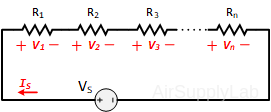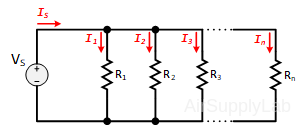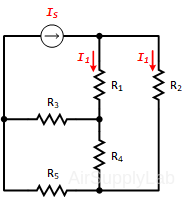# Lab 09: Divider Rules for Voltage and Current

## Objectives

• Practice measuring voltages and current in series and parallel circuits.
• Verify the divider rules for voltage and current.

## Background

#### Voltage Divider

• In parallel circuits, the voltage across each of the component is always same.
• in series circuits, the voltage drop across a resistor is directly proportional to the resistance of the resistor.Figure 1: A Parallel Circuit with n Resistors

The Voltage Divider Rule (VDR) states that the voltage across an element or across a series combination of elements in a series circuit is equal to the resistance of the element or series combination of elements divided by the total resistance of the series circuit and multiplied by the total impressed voltage:#### Current Divider

• In series circuits, the current always remains same through all components.
• In parallel circuits, the current doesn't remains same, instead it divides.Figure 2: A Parallel Circuit with n Resistors

The Current Divider Rule (CDR) states that the current through one of the parallel branches is equal to the resistance of the other branch divided by the sum of the resistances of the two parallel branches and multiplied by the total current entering the parallel branches. That is:## Questions

1. Can you apply current division to obtain I1 and I2 for the circuit shown in the figure below? Explain briefly.